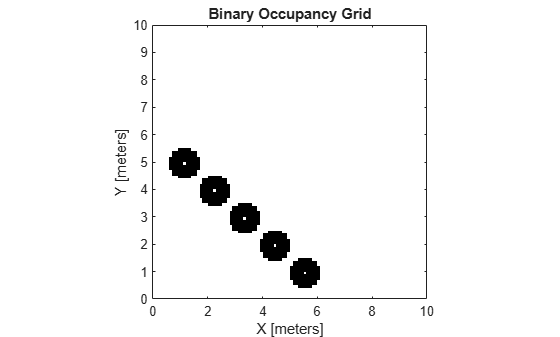Main Content

# setOccupancy

Set occupancy value of locations

## Syntax

``setOccupancy(map,xy,occval)``
``setOccupancy(map,xy,occval,"local")``
``setOccupancy(map,ij,occval,"grid")``
``validPts = setOccupancy(___)``
``setOccupancy(map,bottomLeft,inputMatrix)``
``setOccupancy(map,bottomLeft,inputMatrix,"local")``
``setOccupancy(map,topLeft,inputMatrix,"grid")``

## Description

example

````setOccupancy(map,xy,occval)` assigns occupancy values, `occval`, to the input array of world coordinates, `xy` in the occupancy grid, `map`. Each row of the array, `xy`, is a point in the world and is represented as an `[x y]` coordinate pair. `occval` is either a scalar or a single column array of the same length as `xy` . An occupied location is represented as `true` (`1`), and a free location is represented as `false` (`0`).```
````setOccupancy(map,xy,occval,"local")` assigns occupancy values, `occval`, to the input array of local coordinates, `xy`, as local coordinates.```
````setOccupancy(map,ij,occval,"grid")` assigns occupancy values, `occval`, to the input array of grid indices, `ij`, as `[rows cols]`.```
````validPts = setOccupancy(___)` outputs an `n`-element vector of logical values indicating whether input coordinates are within the map limits.```
````setOccupancy(map,bottomLeft,inputMatrix)` assigns a matrix of occupancy values by specifying the bottom-left corner location in world coordinates.```
````setOccupancy(map,bottomLeft,inputMatrix,"local")` assigns a matrix of occupancy values by specifying the bottom-left corner location in local coordinates.```
````setOccupancy(map,topLeft,inputMatrix,"grid")` assigns a matrix of occupancy values by specifying the top-left cell index in grid indices and the matrix size.```

## Examples

collapse all

Create a 10m x 10m empty map.

`map = binaryOccupancyMap(10,10,10);`

Set occupancy of world locations and show map.

```x = [1.2; 2.3; 3.4; 4.5; 5.6]; y = [5.0; 4.0; 3.0; 2.0; 1.0]; setOccupancy(map, [x y], ones(5,1)) figure show(map)```Inflate occupied locations by a given radius.

```inflate(map, 0.5) figure show(map)```Get grid locations from world locations.

`ij = world2grid(map, [x y]);`

Set grid locations to free locations.

```setOccupancy(map, ij, zeros(5,1), 'grid') figure show(map)```## Input Arguments

collapse all

Map representation, specified as a `binaryOccupancyMap` object. This object represents the environment of the robot. The object contains a matrix grid with binary values indicating obstacles as `true` (`1`) and free locations as `false` (`0`).

World coordinates, specified as an n-by-2 vertical array of ```[x y]``` pairs, where n is the number of world coordinates.

Data Types: `double`

Grid positions, specified as an n-by-2 vertical array of `[i j]` pairs in `[rows cols]` format, where n is the number of grid positions.

Data Types: `double`

Occupancy values of the same length as either `xy` or `ij`, returned as an n-by-1 vertical array, where n is the same n in either `xy` or `ij`. Values are given between 0 and 1 inclusively.

Occupancy values, specified as a matrix. Values are given between 0 and 1 inclusively.

Location of bottom left corner of output matrix in world or local coordinates, specified as a two-element vector, ```[xCoord yCoord]```. Location is in world or local coordinates based on syntax.

Data Types: `double`

Location of top left corner of grid, specified as a two-element vector, `[iCoord jCoord]`.

Data Types: `double`

## Output Arguments

collapse all

Valid map locations, returned as an n-by-1 column vector equal in length to `xy` or `ij`. Locations inside the map return a value of 1. Locations outside the map limits return a value of 0.

## See Also

| | (Navigation Toolbox)

Introduced in R2015a